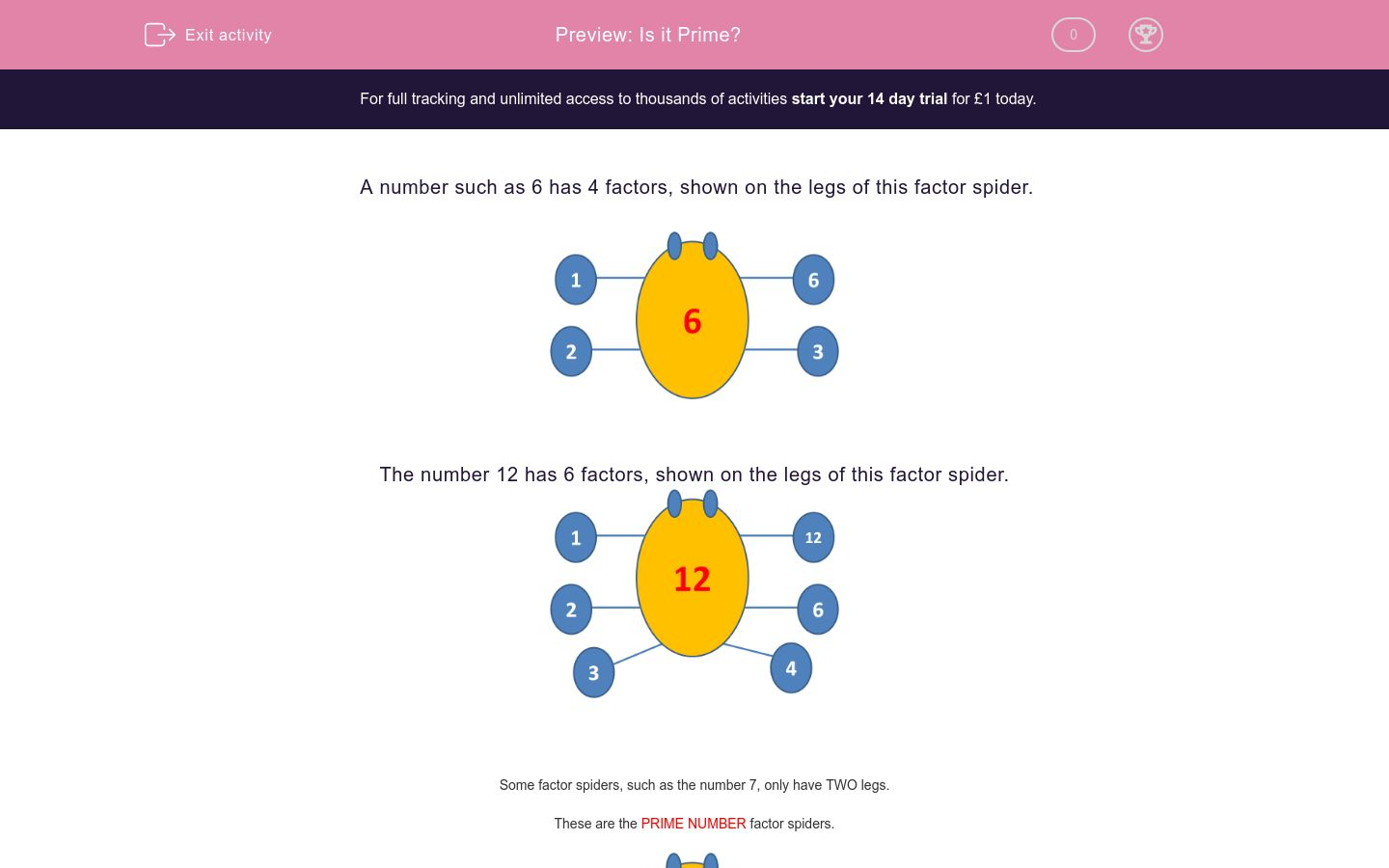# Is it Prime?

In this worksheet, students state whether or not a number is prime.Key stage:  KS 2

Curriculum topic:  Maths and Numerical Reasoning

Curriculum subtopic:  Special Numbers

Difficulty level:\$appendString = '&new=1'; \$_SESSION['guest_aId'] = rand(); \$urlToRedirect = FRAMEWORK_PATH . 'preview/worksheet/introduction?aId=' . \$_SESSION['guest_aId'] . '&wId=' . \$wsData['id'] . '&usertype=guest' . \$appendString; ?>

### QUESTION 1 of 10

A number such as 6 has 4 factors, shown on the legs of this factor spider.The number 12 has 6 factors, shown on the legs of this factor spider.Some factor spiders, such as the number 7, only have TWO legs.

These are the PRIME NUMBER factor spiders.Prime numbers are very special.

They have only two factors, 1 and the number itself.

Is the following number a prime number?

4

Yes

No

Is the following number a prime number?

6

Yes

No

Is the following number a prime number?

12

Yes

No

Is the following number a prime number?

7

Yes

No

Is the following number a prime number?

3

Yes

No

Is the following number a prime number?

9

Yes

No

Is the following number a prime number?

2

Yes

No

Is the following number a prime number?

10

Yes

No

Is the following number a prime number?

11

Yes

No

Is the following number a prime number?

1

Yes

No

• Question 1

Is the following number a prime number?

4

No
• Question 2

Is the following number a prime number?

6

No
• Question 3

Is the following number a prime number?

12

No
• Question 4

Is the following number a prime number?

7

Yes
• Question 5

Is the following number a prime number?

3

Yes
• Question 6

Is the following number a prime number?

9

No
• Question 7

Is the following number a prime number?

2

Yes
EDDIE SAYS
2 is the only even prime number
• Question 8

Is the following number a prime number?

10

No
• Question 9

Is the following number a prime number?

11

Yes
• Question 10

Is the following number a prime number?

1

No
EDDIE SAYS
1 only has one factor. A prime number must have exactly two factors.
---- OR ----

Sign up for a £1 trial so you can track and measure your child's progress on this activity.

### What is EdPlace?

We're your National Curriculum aligned online education content provider helping each child succeed in English, maths and science from year 1 to GCSE. With an EdPlace account you’ll be able to track and measure progress, helping each child achieve their best. We build confidence and attainment by personalising each child’s learning at a level that suits them.

Get startedStart your £1 trial today.
Subscribe from £10/month.